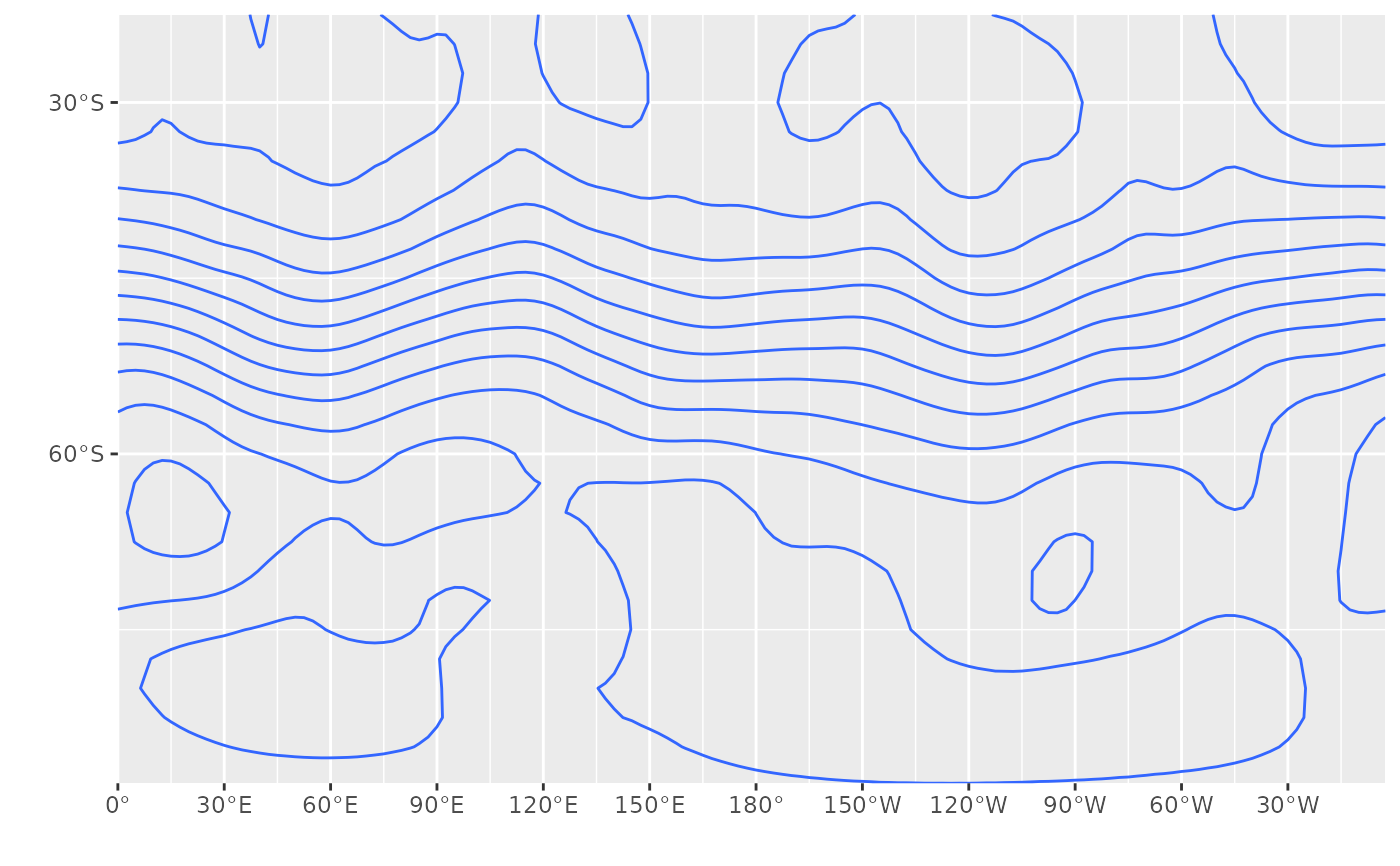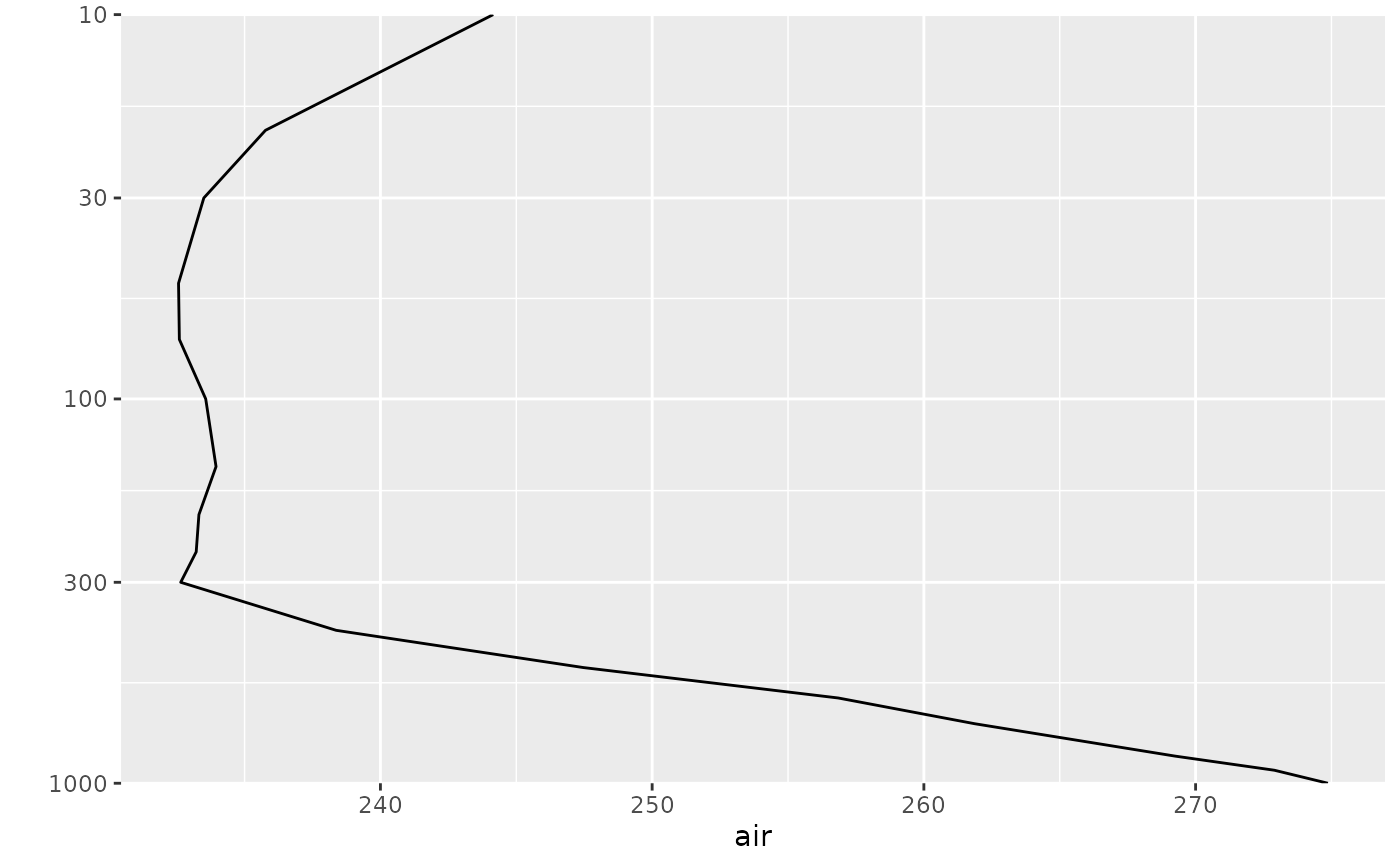These functions are simple wrappers around scale_x_continuous and scale_y_continuous with helpful defaults for plotting longitude, latitude and pressure levels.

scale_x_longitude(
name = "",
ticks = 30,
breaks = seq(-180, 360, by = ticks),
expand = c(0, 0),
labels = LonLabel,
trans = "identity",
...
)

scale_y_longitude(
name = "",
ticks = 60,
breaks = seq(-180, 360, by = ticks),
expand = c(0, 0),
labels = LonLabel,
trans = "identity",
...
)

scale_x_latitude(
name = "",
ticks = 30,
breaks = seq(-90, 90, by = ticks),
expand = c(0, 0),
labels = LatLabel,
...
)

scale_y_latitude(
name = "",
ticks = 30,
breaks = seq(-90, 90, by = ticks),
expand = c(0, 0),
labels = LatLabel,
...
)

scale_x_level(name = "", expand = c(0, 0), trans = "reverselog", ...)

scale_y_level(name = "", expand = c(0, 0), trans = "reverselog", ...)

## Arguments

name

The name of the scale. Used as the axis or legend title. If waiver(), the default, the name of the scale is taken from the first mapping used for that aesthetic. If NULL, the legend title will be omitted.

ticks

spacing between breaks

breaks

One of:

• NULL for no breaks

• waiver() for the default breaks computed by the transformation object

• A numeric vector of positions

• A function that takes the limits as input and returns breaks as output (e.g., a function returned by scales::extended_breaks()). Also accepts rlang lambda function notation.

expand

For position scales, a vector of range expansion constants used to add some padding around the data to ensure that they are placed some distance away from the axes. Use the convenience function ggplot2::expansion() to generate the values for the expand argument.

labels

One of:

• NULL for no labels

• waiver() for the default labels computed by the transformation object

• A character vector giving labels (must be same length as breaks)

• An expression vector (must be the same length as breaks). See ?plotmath for details.

• A function that takes the breaks as input and returns labels as output. Also accepts rlang lambda function notation.

trans

For continuous scales, the name of a transformation object or the object itself. Built-in transformations include "asn", "atanh", "boxcox", "date", "exp", "hms", "identity", "log", "log10", "log1p", "log2", "logit", "modulus", "probability", "probit", "pseudo_log", "reciprocal", "reverse", "sqrt" and "time".

A transformation object bundles together a transform, its inverse, and methods for generating breaks and labels. Transformation objects are defined in the scales package, and are called <name>_trans (e.g., scales::boxcox_trans()). You can create your own transformation with scales::trans_new().

...

Other arguments passed on to scale_(x|y)_continuous()

Other ggplot2 helpers: DivideTimeseries(), MakeBreaks(), WrapCircular(), geom_arrow(), geom_contour2(), geom_contour_fill(), geom_label_contour(), geom_relief(), geom_streamline(), guide_colourstrip(), map_labels, reverselog_trans(), scale_divergent, stat_na(), stat_subset()

## Examples

data(geopotential)
library(ggplot2)
ggplot(geopotential[date == date], aes(lon, lat, z = gh)) +
geom_contour() +
scale_x_longitude() +
scale_y_latitude()data(temperature)
ggplot(temperature[lon == lon & lat == lat], aes(air, lev)) +
geom_path() +
scale_y_level()